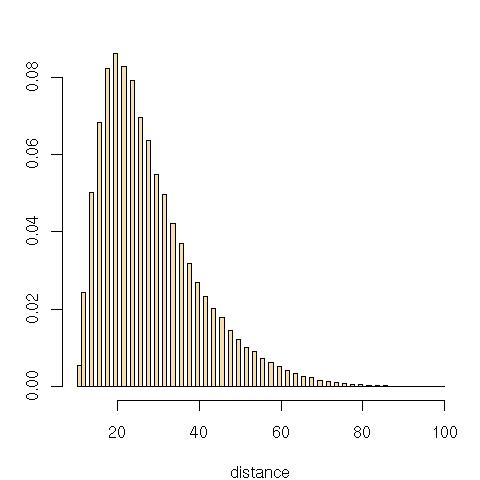Want to share your content on R-bloggers? click here if you have a blog, or here if you don't.

Since my first representation of the rank statistic as paired was incorrect, here is the histogram produced by the simulation

```perm=sample(1:20)
saple[t]=sum(abs(sort(perm[1:10])-sort(perm[11:20])))```

when$n=20$. It is obviously much closer to zero than previously.An interesting change is that the regression of the log-mean on$log(n)$ produces

```> lm(log(memean)~log(enn))
Call:
lm(formula = log(memean) ~ log(enn))
Coefficients:
(Intercept)     log(enn)
-1.162        1.499```

meaning that the mean is in$n^{3/2}$ rather than in$n$ or$n^2$:

```> summary(lm(memean~eth-1))
Coefficients:
Estimate Std. Error t value Pr(>|t|)
eth 0.3117990  0.0002719    1147   <2e-16 ***```

with a very good fit.Filed under: R, Statistics Tagged: Le Monde, puzzle, R, Spearman rank test